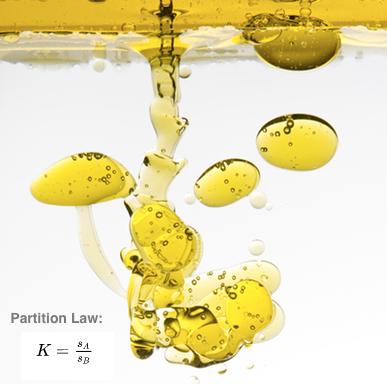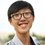# Chemistry - Partition LawProblem Set

What will the scientists do if they want to extract and help purify a desired product from a reaction mixture? This is when the Partition Law(or Distribution Law) saves the day!

First, we set some variables:

Let $A$ and $B$ be two immiscible liquids(cannot dissolve or react with each other), such as water and oil.

Let the solubility of $X$ in liquid $A$ be $s_A$.

Let the solubility of $X$ in liquid $B$ be $s_B$.

Now, we do the following steps:

1) Mix solute $X$ into the mixture of liquid $A$ and $B$.

2) Stir them constantly and wait till liquid $A$ and liquid $B$ are divided into two layers(they will as they cannot dissolve each other).

3) Now, solute $X$ is dissolved in both solvent $A$ and solvent $B$ but the concentration is different.

This is what Partition Law wants to deliver to us.

Definition: At a fixed temperature, when a solute is added to a system consisting of two solvent, it will distribute itself between the two solvent in proportion to its solubility in each of the two solvent separately.

If we express the definition in formula, it will be

$K=\frac{s_A}{s_B}$

where $K$ is the partition coefficient.

If at $40^\circ C$, the solubility of $X$ is $50g/100g$ water and $100g/100g$ ether, then the partition coefficient

$K=\frac{s_{water}}{s_{ether}}=\frac{50g/100g}{100g/100g}=\frac{1}{2}$.

Now there is an example:

If $1L$ water can dissolve $100g$ organic compound $X$ and I use $1L$ ether to extract it, what is the mass in grams of $X$ are left in the ether layer?

Details:

$K=\frac{s_{H_2O}}{s_{ether}}=\frac{1}{2}$

Solution:

Let the amount extracted be $x$ $g$.

The solubility of $X$ in water is $s_{H_2O}=\frac{(100-x)g}{1L}$

The solubility of $X$ in ether is $s_{ether}=\frac{xg}{1L}$

Then,

$K=\frac{s_{H_2O}}{s_{ether}}$

$\frac{1}{2}=\frac{\frac{(100-x)g}{1L}}{\frac{(x)g}{1L}}$

$x=66.7$ $g$

So, the mass of $X$ that left in the ether layer is $66.7$ $g$.

**Note that I use $g/L$ as the unit of solubility instead of $g/g$ in my note before.

Solubility of $5g/1L$ water means $1L$ water can dissolve a maximum of $5g$ of that solute.

Solubility of $5g/100g$ water means $100g$ water can dissolve a maximum of $5g$ of that solute.

So, the unit does not make any changes to the answer as long as we use the same unit. As the example above, we cannot use a unit of solubility written as $g/L$ while the other written as $g/g$.Note by Christopher Boo
7 years, 6 months ago

This discussion board is a place to discuss our Daily Challenges and the math and science related to those challenges. Explanations are more than just a solution — they should explain the steps and thinking strategies that you used to obtain the solution. Comments should further the discussion of math and science.

When posting on Brilliant:

• Use the emojis to react to an explanation, whether you're congratulating a job well done , or just really confused .
• Ask specific questions about the challenge or the steps in somebody's explanation. Well-posed questions can add a lot to the discussion, but posting "I don't understand!" doesn't help anyone.
• Try to contribute something new to the discussion, whether it is an extension, generalization or other idea related to the challenge.

MarkdownAppears as
*italics* or _italics_ italics
**bold** or __bold__ bold
- bulleted- list
• bulleted
• list
1. numbered2. list
1. numbered
2. list
Note: you must add a full line of space before and after lists for them to show up correctly
paragraph 1paragraph 2

paragraph 1

paragraph 2

[example link](https://brilliant.org)example link
> This is a quote
This is a quote
    # I indented these lines
# 4 spaces, and now they show
# up as a code block.

print "hello world"
# I indented these lines
# 4 spaces, and now they show
# up as a code block.

print "hello world"
MathAppears as
Remember to wrap math in $$ ... $$ or $ ... $ to ensure proper formatting.
2 \times 3 $2 \times 3$
2^{34} $2^{34}$
a_{i-1} $a_{i-1}$
\frac{2}{3} $\frac{2}{3}$
\sqrt{2} $\sqrt{2}$
\sum_{i=1}^3 $\sum_{i=1}^3$
\sin \theta $\sin \theta$
\boxed{123} $\boxed{123}$

Sort by:

Why is $x = 66.7g$?

- 7 years, 6 months ago

$\frac{1}{2}=\frac{100-x}{x}$

$x=200-2x$

$3x=200$

$x=66.7$

- 7 years, 6 months ago

Oh... Thanks

- 7 years, 6 months ago

Thx for the information

- 5 years, 3 months ago

Good Post...

- 7 years, 6 months ago

good post

- 7 years, 6 months ago

nice.. :)

- 7 years, 6 months ago

Peter Taylor-

I like the way you added the problems into the notes. However, I don't know how to add a line between the problem and the note. Can you teach me, please?

- 7 years, 6 months ago

To make a line between things use 3 dashes in a row at the beginning of the line. For instance, this is a picture of the markdown on your problem set in the first note.Imgur

Staff - 7 years, 6 months ago

Thanks!

- 7 years, 6 months ago

I also took the liberty of making you a picture. It's not the best, and it is a little bit of stretch, seeing as the post is mostly about partitioning and not about miscibility. If you don't like it, I can take the picture away.

Staff - 7 years, 6 months ago

The picture is very nice, thanks!

- 7 years, 6 months ago

Thanks Christopher for this post which is completely new to me.

- 7 years, 6 months ago

Thanks for the post! Never learned this back in secondary school.

- 7 years, 6 months ago

What does "extract" mean?

- 7 years, 6 months ago

Literal Definition: to remove/separate a certain substance(useful) from another substance through various Physical and Chemical methods.

- 7 years, 6 months ago

To obtain a substance by chemical or mechanical action, as by pressure, distillation, or evaporation. (Google)

- 7 years, 6 months ago

Thanks

- 7 years, 6 months ago

If 10.0 mg of compound is disolved in 50.0ml of water and this extracted with 25.0 ml of CCL4 . How many mg remain. K=56

- 6 months, 4 weeks ago